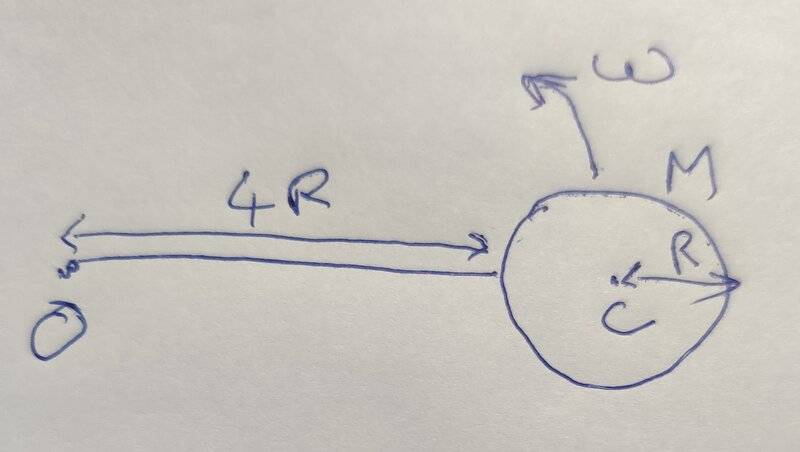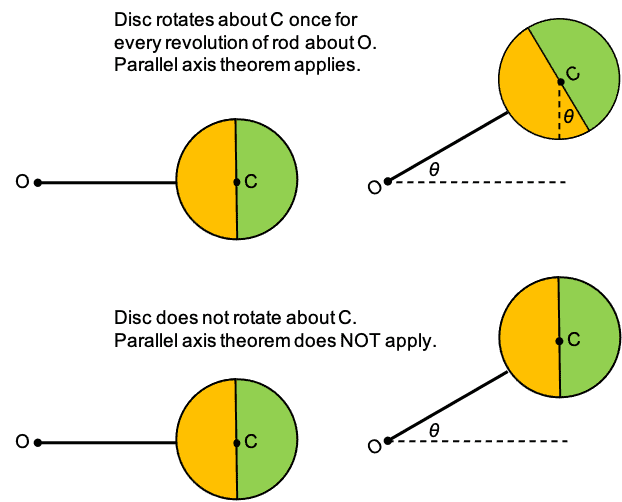# Moment of inertia of a disk about an axis not passing through its CoM

vcsharp2003
Homework Statement:
A disk of radius R and mass M is connected to a rod that is rotating about O. The rod is rotating about at an angular velocity of ##\omega## about O. What would be the moment of inertia of the rod+disk system about an axis through O and perpendicular to plane of rotation of the rod. Assume the rod is massless and the disk is not rotating about it's center of mass axis.
Relevant Equations:
##I=\frac {MR^2} {2}## for a disk about an axis through its cm and perpendicular to the disk
## I_o = I_{cm} + M R^2##
I have come up with two different approaches, but I'm not sure which one is correct since they give different answers.
We use the following equation to get the total moment of inertia.

##I_o## = moment of inertia of disk about O axis + moment of inertia of road about O axis

Approach 1:

Consider the disk as a particle at it's center of mass.
##I_o = M (5R)^2 + 0= 25 MR^2##

Approach 2:

We use parallel axis theorem to get moment of inertia of disk about O axis.

##I_o= \frac {MR^2} {2} + M (5R)^2 + 0 \\= \frac {51MR^2} {2}##Last edited:

Staff Emeritus
Homework Helper
Gold Member
Consider the disk as a particle at it's center of mass.
The disk is not a particle at its center of mass.

vcsharp2003
The disk is not a particle at its center of mass.
If an object is moving at an angular velocity, and its of uniform density then can we not assume it's like a particle at its cm rotating about O in this scenario?

Homework Helper
Gold Member
2022 Award
If an object is moving at an angular velocity, and its of uniform density then can we not assume it's like a particle at its cm rotating about O in this scenario?
No.
E.g. consider a mass 2m at radius r. MoI=2mr2.
Now split it into mass m at radius 0 and mass m at radius 2r. Centre of mass has not changed but MoI=4mr2.

But I note this in post #1: "the disk is not rotating about its center of mass axis."
Technically, that means it retains the same orientation even though its centre rotates about O. I.e. if the rod is rotating at ##\omega## then the disc is rotating at the same rate in the opposite direction relative to the rod. In that case you can treat it as a point particle.
But maybe it means that it is attached rigidly to the rod and so rotates about its own centre at the same rate that it rotates about O. In that case you need to apply the parallel axis theorem.

•vcsharp2003
vcsharp2003
Technically, that means it retains the same orientation even though its centre rotates about O
Yes, it retains its orientation. So, we must assume its a particle.
But still, I cannot explain as to why the parallel axis theorem approach is not correct in this case.

Homework Helper
How does your diagram fits the description "centered at O"? Is this the original text or/and figure?

•vcsharp2003
vcsharp2003
How does your diagram fits the description "centered at O"? Is this the original text or/and figure?
Thanks for pointing out the mistake. I have corrected it so it doesn't say that. This is a made up problem by me in which I am trying to understand the right way to find moment of inertia of a system.

vcsharp2003
Technically, that means it retains the same orientation even though its centre rotates about O. I.e. if the rod is rotating at ω then the disc is rotating at the same rate in the opposite direction relative to the rod. In that case you can treat it as a point particle.
This disk is not rotating about its center of mass axis. It simply moves in a circular orbit and is connected to rod rigidly. Its like a stone tied to the end of a string and the stone is moving in a circular path.

Homework Helper
Gold Member
2022 Award
Yes, it retains its orientation.

and is connected to rod rigidly.
You cannot have it both ways. If it retains its orientation as the rod rotates then it must be mounted on an axle, not rigidly attached.

•vcsharp2003
vcsharp2003
You cannot have it both ways. If it retains its orientation as the rod rotates then it must be mounted on an axle, not rigidly attached.
Ok, I get that. But, why the parallel axis theorem would not be applicable in this problem? I thought it always applies for two parallel axes provided one of the axes is passing through the center of mass of disk.

Homework Helper
Gold Member
2022 Award
Ok, I get that. But, why the parallel axis theorem would not be applicable in this problem? I thought it always applies for two parallel axes provided one of the axes is passing through the center of mass of disk.
Yes, but it only applies if the object is rotating as a body about that parallel axis.
More generally, suppose the mass centre of the body rotates at radius r at rate ##\omega_b## about some point, but the orientation of the body rotates at rate ##\omega_c## about the body's mass centre. Then the angular momentum is ##mr^2\omega_r+I_c\omega_c##. The parallel axis theorem is just the special case where the rotation rates are the same.

•vcsharp2003•